Next: Continuity Equation Up: Basic Equation of Fluid Previous: Equation of Motion   Contents

# Lagrangian and Euler Equations

The time derivative appearing in equation (A.1) expresses how the velocity of a specific particle changes. Therefore, what appears in equation (A.3) means the same, that is, the position of the gas element concerning in equation (A.3) moves and the positions at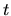and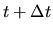are generally different. However, considering the velocity field in the space, the time derivative of the velocity should be calculated staying at a fixed point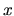.

These two time derivative are different each other and should be distinguished. The former time derivative is called Lagrangian time derivative and is expressed using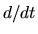. On the other hand, the latter is called Eulerian time derivative and is expressed using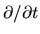. These two are related with each other. Consider a function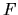whose independent variables are timeand position, that is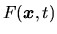. The difference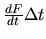, using the Lagrangian time derivative of, represents the the difference of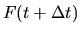from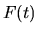focusing on a specific fluid element, whose positions are different owing to its motion. The element at the position of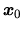at the epoch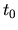moves to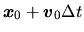in time span of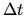. Thus the difference is expressed as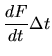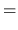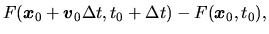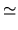(A.4)

where we used the Taylor expansion of. The difference corresponding to the Eulerian derivative is written down as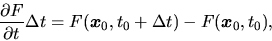(A.5)

and this is equal to the second term of the rhs of equation (A.4). Comparing equations (A.4) and (A.5), the Lagrangian derivative contains an extra term besides the Eulerian derivative. That is, the Lagrangian derivative is expressed by the Eulerian derivative as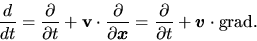(A.6)

Applying the above expression on equation of motion based on the Lagrangian derivative (A.3), we obtain the Eulerian equation motion: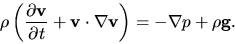(A.7)Next: Continuity Equation Up: Basic Equation of Fluid Previous: Equation of Motion   Contents
Kohji Tomisaka 2007-07-08